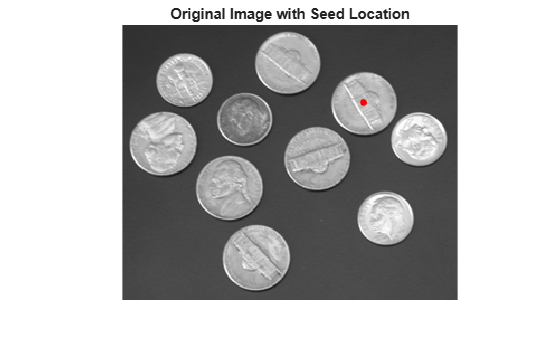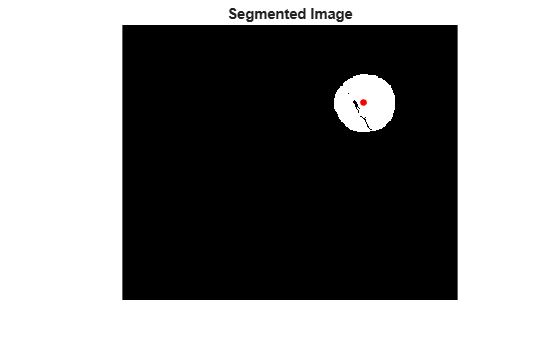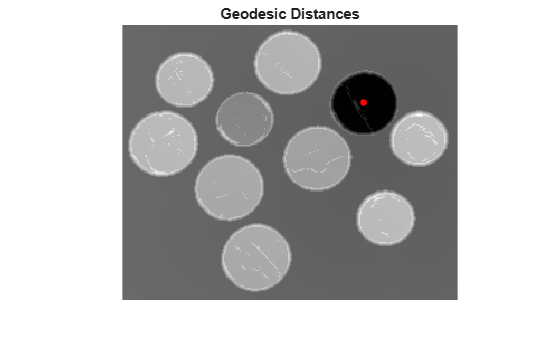Calculate weights for image pixels based on image gradient

Description

W = gradientweight(I) calculates the pixel weight for each pixel in image I based on the gradient magnitude at that pixel, and returns the weight array W. The weight of a pixel is inversely related to the gradient values at the pixel location. Pixels with small gradient magnitude (smooth regions) have a large weight and pixels with large gradient magnitude (such as on the edges) have a small weight.

W = gradientweight(I,sigma) uses sigma as the standard deviation for the derivative of Gaussian that is used for computing the image gradient.

example

W = gradientweight(___,Name,Value) returns the weight array W using name-value pairs to control aspects of weight computation.

Examples

collapse all

This example segments an image using the Fast Marching Method based on the weights derived from the image gradient.

imshow(I)
title('Original Image')Compute weights based on image gradient.

sigma = 1.5;
W = gradientweight(I, sigma, 'RolloffFactor', 3, 'WeightCutoff', 0.25);

Select a seed location.

R = 70; C = 216;
hold on;
plot(C, R, 'r.', 'LineWidth', 1.5, 'MarkerSize',15);
title('Original Image with Seed Location')Segment the image using the weight array.

thresh = 0.1;
[BW, D] = imsegfmm(W, C, R, thresh);
figure, imshow(BW)
title('Segmented Image')
hold on;
plot(C, R, 'r.', 'LineWidth', 1.5, 'MarkerSize',15);Geodesic distance matrix D can be thresholded using different thresholds to get different segmentation results.

figure, imshow(D)
title('Geodesic Distances')
hold on;
plot(C, R, 'r.', 'LineWidth', 1.5, 'MarkerSize',15);Input Arguments

collapse all

Grayscale image, specified as a numeric matrix.

Data Types: single | double | int8 | uint8 | int16 | uint16 | int32 | uint32

Standard deviation for derivative of Gaussian, specified as a positive number.

Data Types: double

Name-Value Arguments

Specify optional comma-separated pairs of Name,Value arguments. Name is the argument name and Value is the corresponding value. Name must appear inside quotes. You can specify several name and value pair arguments in any order as Name1,Value1,...,NameN,ValueN.

Output weight roll-off factor, specified as the comma-separated pair consisting of 'RolloffFactor' and a positive scalar of class double. Controls how fast weight values fall as a function of gradient magnitude. When viewed as a 2-D plot, pixel intensity values might vary gradually at the edges of regions, creating a gentle slope. In your segmented image, you might want the edge to be more well-defined. Using the roll-off factor, you control the slope of the weight value curve at points where intensity values start to change. If you specify a high value, the output weight values fall off sharply around the edges of smooth regions. If you specify a low value, the output weight has a more gradual fall-off around the edges. The suggested range for this parameter is [0.5 4].

Data Types: double

Threshold for weight values, specified as the comma-separated pair consisting of 'WeightCutoff' and a positive number in the range [1e-3 1]. If you use this parameter to set a threshold on weight values, it suppresses any weight values less than the value you specify, setting these pixels to a small constant value (1e-3). This parameter can be useful in improving the accuracy of the output when you use the output weight array W as input to Fast Marching Method segmentation function, imsegfmm.

Data Types: double

Output Arguments

collapse all

Weight array, returned as a numeric array of the same size as the input image, I. The weight array is of class double, unless I is single, in which case it is of class single.

Tips

• gradientweight uses double-precision floating point operations for internal computations for all classes of I, except when I is of class single, in which case gradientweight uses single-precision floating point operations internally.